Core java - Interview Questions and Answers for 'Leidos' | Search Java Interview Question - javasearch.buggybread.com# Search Interview QuestionsMore than 3000 questions in repository.There are more than 900 unanswered questions.Have a video suggestion.
Click Correct / Improve and please let us know.
Label / Company      Label / Company / TextSubmit Question

Core java - Interview Questions and Answers for 'Leidos' - 2 question(s) found - Order By Newest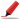Very frequently asked. Among first few questions in almost all interviews. Among Top 5 frequently asked questions. Frequently asked in Indian service companies (HCL,TCS,Infosys,Capgemini etc based on multiple feedback )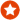Q1. Difference between == and .equals() ?Core Java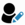Anonymous
Ans. "equals" is the method of object class which is supposed to be overridden to check object equality, whereas "==" operator evaluate to see if the object handlers on the left and right are pointing to the same object in memory.

x.equals(y) means the references x and y are holding objects that are equal. x==y means that the references x and y have same object.

Sample code:

String x = new String("str");
String y = new String("str");

System.out.println(x == y); // prints false
System.out.println(x.equals(y)); // prints true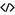Sample Code for equalsHelp us improve. Please let us know the company, where you were asked this question :LikeDiscussCorrect / Improvejava   string comparison   string   object class   ==    equals   object equality  operator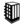Asked in 292 Companies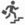basic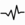frequent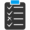Try 6 Question(s) TestRelated Questions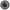What is the difference between = and == in Java ?How is == operator different for objects and primitive types ?What will be the output of following code

Integer x = 1;
Integer y = 2;
System.out.println(x == y);

What if you change 1 to "1" and Integer to String?Frequently asked in face to face interviews.Q2. Write a program to print fibonacci series.Core JavaAns. int count = 15;
int[] fibonacci = new int[count];
fibonacci = 0;
fibonacci = 1;
for(int x=2; x < count; x++){
fibonacci[x] = fibonacci[x-1] + fibonacci[x-2];
}

for(int x=0; x< count; x++){
System.out.print(fibonacci[x] + " ");
}Help us improve. Please let us know the company, where you were asked this question :LikeDiscussCorrect / Improveebay   fibonacci seriesAsked in 66 CompaniesbasicfrequentRelated QuestionsWhat are the steps to be performed while coding Junit with Mocking framework ?What is a Sequence File?What different level of logging you use while coding ?Have you ever encoded the response before sending it back from the service? If Yes , Which encoding was used ?What are the different type of encoding you have used ?How does encoding affect using Reader / writer classes or Stream classes in Java ?What is the difference between html encoding and url encoding ?Does spaces get's encoded in html encoding ?

## Help us and Others Improve. Please let us know the questions asked in any of your previous interview.

Any input from you will be highly appreciated and It will unlock the application for 10 more requests.